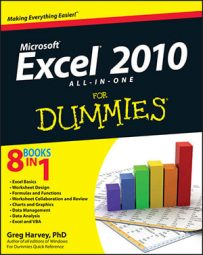##### Excel 2010 All-in-One For DummiesExcel 2010 includes several time functions that you can use in your worksheets. Use the HOUR, MINUTE, and SECOND time functions to extract the hours, minutes, and seconds from a single time serial number.

The HOUR, MINUTE, and SECOND functions let you extract specific parts of a time value in the worksheet. Each of these three time functions takes a single serial_number argument that contains the hour, minute, or second that you want to extract.

So, for example, if cell B5 contains the time 1:30:10 PM (otherwise known as serial number 0.5626157) and you enter the following HOUR function in cell C5:

`=HOUR(B5)`

Excel returns 13 as the hour to cell C5 (hours are always returned in 24-hour time). If you then enter the following MINUTE function in cell D5:

`=MINUTE(B5)`

Excel returns 30 as the number of minutes to cell D5. Finally, if you enter the following SECOND function in cell E5:

`=SECOND(B5)`

Excel returns 10 as the number of seconds to cell E5.